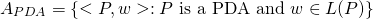# Decidable Languages : ACFG, APDA

A language is decidable if there is a Turing Machine that halts and accepts strings that belong to the language, and halts and rejects strings that do not belong to the language.

## ACFG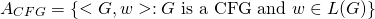There exists a TM that decides the above language. Given the string encoding of a CFG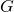and some string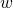, a TM can decide whethercan derive.

## Greibach Normal Form (GNF)

Any CFG can be converted to Greibach Normal Form. In this form, all production rules start with a terminal symbol, optionally followed by some variables. The only exception is the start variable which can go to.

Formally, a CFG is in Greibach normal form, if all production rules are of the form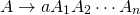or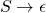where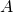is a nonterminal symbol,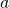is a terminal symbol,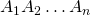is a (possibly empty) sequence of nonterminal symbols not including the start symbol,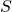is the start symbol, andis the empty word.

One interesting property about a grammar in this form is related to parsing. Given a grammar in GNF and a derivable string in the grammar with length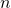, any top-down parser will halt at depth.

## High-Level Description

On input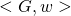1. Check whether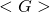indeed encodes a CFG. If not, reject.
2. Convertto a CFG,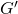in GNF.
3. If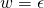, check if the rule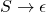is present in. If yes, accept. Else reject.
4. Let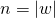.
5. Examine all derivations usingapplications of rules in. If any derivation producesaccept. Else reject.

Since all these steps take a finite amount of time, it is guaranteed that the machine halts and decides.

Any PDA can be converted to an equivalent CFG. Thus, the following language is also decidable since it reduces to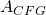.

## APDA